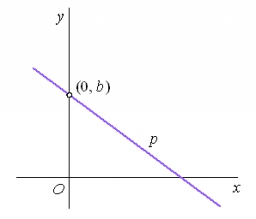# Half-planes 36831

The line p and the two inner points of one of the half-planes determined by the line p are given. Find point X on the line p so that the sum of its distances from points A and B is the smallest.

x =  1

### Step-by-step explanation:Did you find an error or inaccuracy? Feel free to write us. Thank you!

Tips for related online calculators
The line slope calculator is helpful for basic calculations in analytic geometry. The coordinates of two points in the plane calculate slope, normal and parametric line equation(s), slope, directional angle, direction vector, the length of the segment, intersections of the coordinate axes, etc.
Do you want to convert length units?